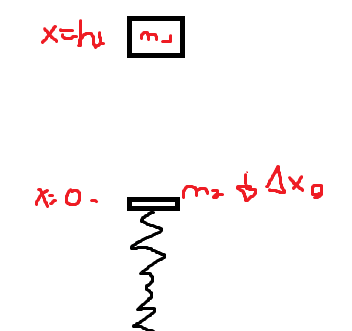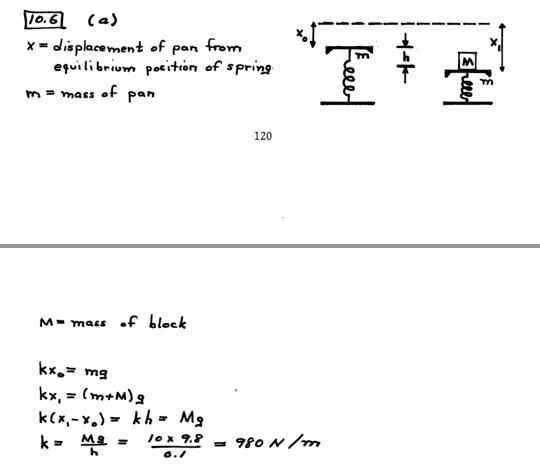# Find the spring constant k

LCSphysicist
Homework Statement:
A mass of 10 kg falls 50 cm onto the platform of a spring scale,
and sticks. The platform eventually comes to rest 10 cm below its initial
position. The mass of the platform is 2 kg. Find the spring constant.
Relevant Equations:
All belowThis is my scope of the question, i could think to solve it by two steps, but before, let's give name to the things.

X is positive down direction.
X = 0 at the initial position o the platform
Mass of the falling block is m1
Mass of the platform, m2
Spring constant k
Δx is the initial stretched length of the spring
h1 is the height of the block
h2 is the final distance (0,1m) of the origin
vb is the block speed
vc is the both bodies speed

vb² = 2gh1
m1*vb = (m1+m2)*vc
(m1+m2)*vc²/2 + kΔx²/2 = -(m1+m2)*g*|h2| + k(Δx + h2)²/2
Δx = m2*g/k

That is a system possible and determined, but why this is wrong?
I see we could apply kx before and in the final scenario, but why the first attempt is wrong?

Homework Helper
Gold Member
2022 Award
The question is not entirely clear. It says "eventually " comes to rest. That suggests it is at the new equilibrium position, so the initial drop is irrelevant.
You have interpreted it as "first" comes to rest. Under that view, your work looks correct to me. How do you know it is considered wrong? Do you get a numerical answer different from a given one? If so, what are the two numbers?

•Merlin3189
LCSphysicist
The question is not entirely clear. It says "eventually " comes to rest. That suggests it is at the new equilibrium position, so the initial drop is irrelevant.
You have interpreted it as "first" comes to rest. Under that view, your work looks correct to me. How do you know it is considered wrong? Do you get a numerical answer different from a given one? If so, what are the two numbers?
HI :D I have no a numerical answer, but there is this solution on the internetI think this is correct too, since the question says that in both cases the spring is in rest. If this is right, i agree that my answer would need too, but solving leads to differing values.